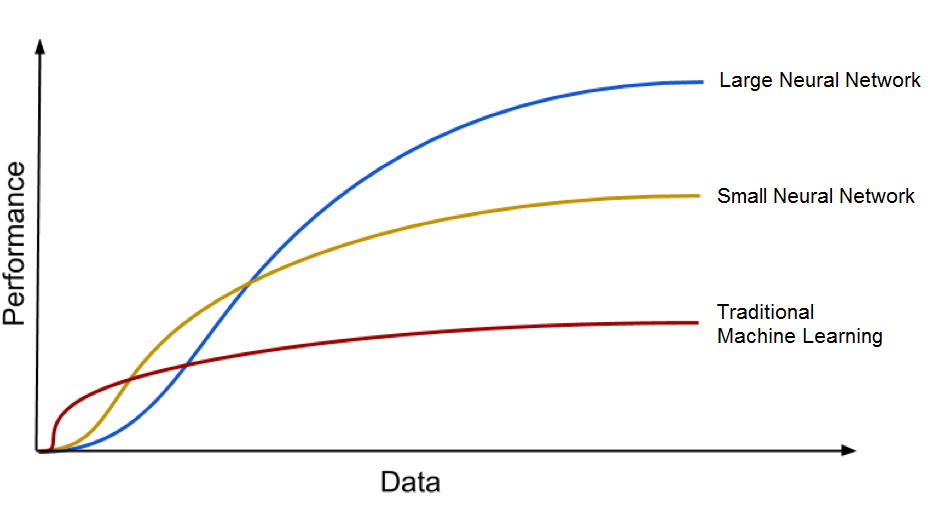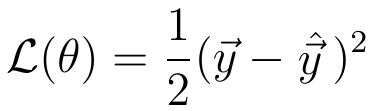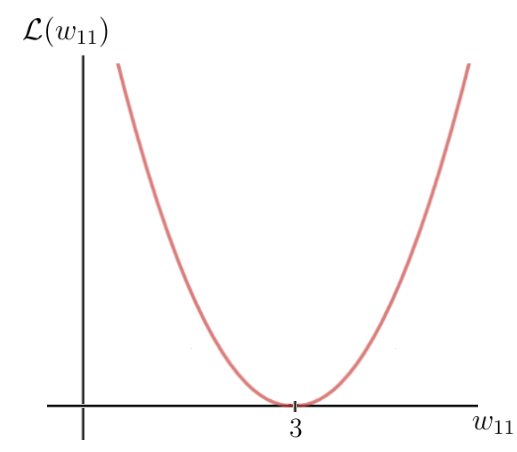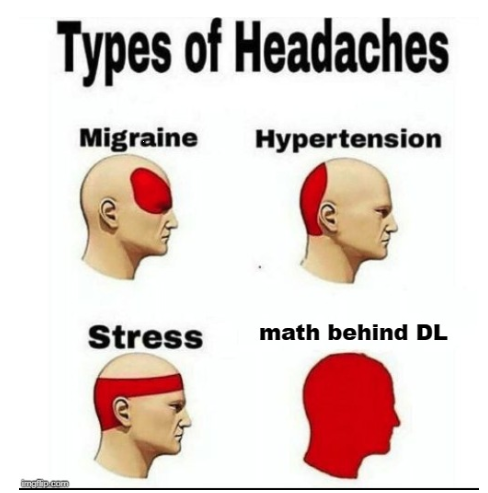This is a beginner's guide to Deep Learning and Neural networks. In the following article, we are going to discuss the meaning of Deep Learning and Neural Networks. In particular, we will focus on how Deep Learning works in practice.

Have you ever wondered how Google’s translator App is able to translate entire paragraphs from one language into another in a matter of milliseconds? How Netflix and YouTube are able to figure out our taste in movies or videos and give us appropriate recommendations? Or how self-driving cars are even possible?All of this is a product of Deep Learning and Artificial Neural Networks. The definition of Deep Learning and Neural networks will be addressed in the following. Lets us begin with Deep Learning first.

## 1. What exactly is Deep Learning?

Deep Learning is a subset of Machine Learning, which on the other hand is a subset of Artificial Intelligence. Artificial Intelligence is a general term that refers to techniques that enable computers to mimic human behavior. Machine Learning represents a set of algorithms trained on data that make all of this possible.

Deep Learning, on the other hand, is just a type of Machine Learning, inspired by the structure of a human brain. In terms of Deep Learning this structure is called an Artificial Neural Network. (For a more detailed explanation of the difference between Deep Learning, Machine Learning and AI please refers to this blog article.)

Neural networks are a set of algorithms are designed to recognize patterns in the data. They interpret sensory data through a kind of machine perception, labeling or clustering raw input. The patterns that are recognized by neural networks are numeric, contained in vectors, into which all real-world data, like text, time series, images or sound must be translated.

Neural networks enable us to perform many tasks, such as clustering, classification or regression. With neural networks, we can group or sort unlabeled data according to similarities among the samples in this data. Or in the case of classification, we can train the network on a labeled dataset in order to classify the samples in this dataset into different categories.

Let us take a look at a classification example and see how a machine learning model would handle this task in comparison to a deep learning model. Please assume that we create an algorithmic model that can differentiate between images of handwritten numbers. Or in other words: that can classify a handwritten number into the categories of 0–9:

If we would use a Machine Learning model, it would be necessary to tell the model the features on which the numbers can be differentiated. These features could be for examples the shape of a particular number. With Deep Learning, on the other hand, the features are learned by the neural network — without human intervention.

To achieve this kind of independence neural networks require much more data than typical Machine Learning algorithms. However, another major advantage of neural networks over machine learning is that neural networks can achieve a much better performance than their machine learning counterparts.## 2. Biological Neural Networks

Before we move any further with artificial neural networks I would like to introduce the concept behind biological neural networks, so when we will later discuss the artificial neural network in more detail we can see parallels with the biological model.

Artificial neural networks are inspired by the biological neurons that are found in our brains. In fact, the artificial neural networks simulate some basic functionalities of the neural networks in our brain, but in a very simplified way. Let’s first look at the biological neural networks to derive parallels to artificial neural networks. In short, a biological neural network consists of numerous neurons.

A typical neuron consists of a cell body, dendrites, and an axon. Dendrites are thin structures that emerge from the cell body. An axon is a cellular extension that emerges from this cell body. Most neurons receive signals through the dendrites and send out signals along the axon.

At the majority of synapses, signals cross from the axon of one neuron to the dendrite of another. All neurons are electrically excitable due to the maintenance of voltage gradients in their membranes. If the voltage changes by a large enough amount over a short interval, the neuron generates an electrochemical pulse called an action potential. This potential travels rapidly along the axon and activates synaptic connections as it reaches them.

In this way, the neurons can communicate with each other across the entire network. The learning process in a human brain is not yet fully understood, but scientists are pretty sure that the learning process can be explained by changing the strength of connections between neurons.

Neurons that exchange action potential more often have stronger axon connections between each other and vise versa. Tasks we as humans learn and new experiences we gain, force this alteration of connection between neurons. Some neurons become more interconnected, some less. Some connections may even completely die off. In order for us humans to solve a specific task, the biological neural network in our brain has to develop certain neuronal connections in which some neurons are more interconnected and others less interconnected.

## 3. Artificial Neural Networks

Now that we have a basic understanding of how biological neural networks are functioning, let’s finally take a look at the architecture of the artificial neural network.

A neural network generally consists of a collection of connected units or nodes. We call these nodes neurons. These artificial neurons loosely model the biological neurons of our brain.

A neuron is simply a graphical representation of a numeric value (e.g. 1.2, 5.0, 42.0, 0.25, etc.). Any connection between two artificial neurons can be considered as an axon in a real biological brain.

The connections between the neurons are realized by so-called weights, which are also nothing more than numerical values

The weights between the neurons determine the learning ability of the neural network. As mentioned before in biological neural networks, learning can be explained by alteration of the strength of the connection between neurons. The same principle applies to an artificial neural network.

When an artificial neural network learns, the weights between neurons are changing and so does the strength of the connection Meaning: Given training data and a particular task such as classification of numbers, we are looking for certain set weights that allow the neural network to perform the classification. The set of weights is different for every task and every dataset. We can not predict the values of these weights in advance, but the neural network has to learn them. The process of learning we also call as training.

## 4. Typical Neural Network Architecture

The typical neural network architecture consists of several layers. We call the first layer as the input layer. The input layer receives the input x, data from which the neural network learns. In our previous example of classification of handwritten numbers, these input x would represent the images of these numbers (x is basically an entire vector where each entry is a pixel). The input layer has the same number of neurons as there are entries in the vector x. Meaning: each input neuron represents one element in the vector x.

The last layer is called the output layer, which outputs a vector y representing the result that the neural network came up with. The entries in this vector represent the values of the neurons in the output layer. In our case of classification, each neuron in the last layer would represent a different class. In this case, a value of an output neuron gives the probability that the handwritten digit given by the features x belongs to one of the possible classes (one of the digits 0–9). As you can imagine the number of output neurons must be the same as there are classes.

In order to obtain a prediction vector y, the network must perform certain mathematical operations. These operations are performed in the layers between the input and output layers. We call these layers the hidden layers. Now lets us discuss how the connections between the layers look like.

## 5. Layer Connections in a Neural Network

Please consider a smaller example of a neural network that consists of only two layers. The input layer has two input neurons, while the output layer consists of three neurons.

As mentioned earlier, each connection between two neurons is represented by a numerical value, which we call weight. As you can see in the picture, each connection between two neurons is represented by a different weight w. Each of these weights w has indices. The first value of the indices stands for the number of neurons in the layer from which the connection originates, the second value for the number of the neurons in the layer to which the connection leads.

This means that each indice represents the two neurons that are interconnected. For example, the weight w23 realizes the connection between the second neuron of the first layer and the third neuron of the second layer.

All weights between two neural network layers can be represented by a matrix called the weight matrix.

As you know, each entry in any matrix contains indexes based on the position of that entry in a particular row and column. The entries of the weight matrix represent the weight values with the corresponding indices.

A weight matrix has the same number of entries as there are connections between neurons. The dimensions of a weight matrix result from the sizes of the two layers that are connected by this weight matrix. The number of rows corresponds to the number of neurons in the layer from which the connections originate and the number of columns corresponds to the number of neurons in the layer to which the connections lead.

In this particular example, the number of rows of the weight matrix corresponds to the size of the input layer which is two and the number of columns to the size of the output layer which is three.

## 6. Learning Process of a Neural Networks

Now that we understand the neural network architecture better, we can intuitively study the learning process. Let us do it step by step. The first step is already known to you. For a given input feature vector x, the neural network calculates a prediction vector, which we call here as h.

This step is also referred to as the forward propagation. With the input vector x and the weight matrix W connecting the two neuron layers, we compute the dot product between the vector x and the matrix W.

The result of this dot product is again a vector, which we call z. The final prediction vector h is obtained by applying a so-called activation function to the vector z. In this case, the activation function is represented by the letter Sigma. An activation function is only a nonlinear function that performs a nonlinear mapping from z to h. There are 3 activation functions that are used in Deep Learning, which are tanh, sigmoid and ReLu.

At this point, you may recognize the meaning behind neurons in a neural network. A neuron is simply a representation of a numeric value.

The neurons of the input layers represent the values of the input features x. The neurons of the output layer represent the predictions that the neural network calculated. The values of the neurons in the hidden layer are basically just some intermediate values that are used for the calculation.

Let’s take a closer look at vector z for a moment. As you can see, each element of z consists of the input vector x. At this point, the role of the weights unfolds beautifully. A value of a neuron in a layer consists of a linear combination of neuron values of the previous layer weighted by some numeric values. These numerical values are the weights that tell us how strongly these neurons are connected with each other.

During training, these weights are adjusted, some neurons become more connected, some neurons become less connected. As in a biological neural network, learning means the alteration of weights. Accordingly, the values of z, h and the final output vector y are changing with the weights. Some weights make the predictions of a neural network us closer to the actual ground truth vector y_hat, some weights increase the distance to the ground truth vector.

Now that we know how the mathematical calculations between two neural network layers look like, we can extend our knowledge to a deeper architecture that consists of 5 layers.

Same as before we calculate the dot product between the input x and the first weight matrix W1 and apply an activation function to the resulting vector to obtain the first hidden vector h1. h1 is now considered as the input for the upcoming third layer. The whole procedure from before is repeated until we obtain the final output y:

## 7. Loss Functions

After we get the prediction of the neural network, in the second step we must compare this prediction vector to the actual ground true label. We call the ground truth label as vector y_hat. While the vector y contains the predictions that the neural network has computed during the forward propagation (and which may, in fact, be very different from the actual values), the vector y_hat contains the actual values.

Mathematically, we can measure the difference between y and y_hat by defining a loss function which value depends on this difference.

An example of a general loss function is the quadratic loss:Since the prediction vector y is a function of the weights of the neural network (which we abbreviate to theta), the loss is also a function of the weights.

The value of this loss function depends on the difference between y_hat and y. A higher difference means a higher loss value, a smaller difference means a smaller loss value. Minimizing the loss function directly leads to more accurate predictions of the neural network, as the difference between the prediction and the label decreases.

In fact, the minimization of the loss function is the only objective that the neural network tries to achieve. Remember when I said that the neural network solves tasks without being explicitly programmed with a task-specific rule. This is possible because minimizing the loss function as a goal is universal and does not depend on the task or task circumstances.

Minimizing the loss function automatically causes the neural network model to make better predictions regardless of the exact characteristics of the task at hand. You only have to select the right loss function for the task. Fortunately, there are only two loss functions that you should know about to solve almost any problem that you encounter in practice.

These loss-functions are the Cross-Entropy Loss:and the Mean Squared Error Loss:Since the loss depends on the weights, we must find a certain set of weights for which the value of the loss function is as small as possible. The method of minimizing the loss function is achieved mathematically by a method called gradient descent.

During gradient descent, we use the gradient of a loss function (or in other words the derivative of the loss function) to improve the weights of a neural network.

To understand the basic concept of the gradient descent process, let us consider a very basic example of a neural network consisting of only one input and one output neuron connected by a weight value w.This neural network receives an input x and outputs a prediction y. Let say the initial weight value of this neural network is 5 and the input x is 2. Therefore the prediction y of this network has a value of 10, while the label y_hat might have a value of 6.This means that the prediction is not accurate and we must use the gradient descent method to find a new weight value that causes the neural network to make the correct prediction. In the first step, we must choose a loss function for the task. Let’s take the quadratic loss that I have defined earlier and plot this function, which basically is just a quadratic function:The y-axis is the loss value which depends on the difference between the label and the prediction, and thus the network parameters, in this case, the one weight w. The x-axis represents the values for this weight. As you can see there is a certain weight w for which the loss function reaches a global minimum. This value is the optimal weight parameter that would cause the neural network to make the correct prediction which is 6. In this case, the value for the optimal weight would be 3:Our initial weight, on the other hand, is 5, which leads to a fairly high loss. The goal now is to repeatedly update the weight parameter until we reach the optimal value for that particular weight. This is the time when we need to use the gradient of the loss function. Fortunately, in this case, the loss function is a function of one single variable, which is the weight w:In the next step, we calculate the derivative of the loss function with respect to this parameter:In the end, we get a result of 8, which gives us the value of the slope or the tangent of the loss function for the corresponding point on the x-axis at which our initial weight lies.

This tangent points towards the highest rate of increase of the loss function and the corresponding weight parameters on the x-axis.

This means that we have just used the gradient of the loss function to find out which weight parameters would result in an even higher loss value. But what we want to know is the exact opposite. We can get what we want, if we multiply the gradient by minus 1 and this way obtain the opposite direction of the gradient. This way we get the direction of the highest rate of decrease of the loss function and the corresponding parameters on the x-axis that cause this decrease:In the final step, we perform one gradient descent step as an attempt to improve our wights. We use this negative gradient to update your current weight in the direction of the weights for which the value of the loss function decreases according to the negative gradient:The factor epsilon in this equation is a hyperparameter called the learning rate. The learning rate determines how quickly or how slowly you want to update the parameters. Please keep in mind that the learning rate is the factor with which we have to multiply the negative gradient and that the learning rate is usually quite small. In our case, the learning rate is 0.1.

As you can see, our weight w after the gradient descent is now 4.2 and closer to the optimal weight than it was before the gradient step.The value of the loss function for the new weight value is also smaller, which means that the neural network is now capable to do a better prediction. You can do the calculation in your head and see that the new prediction is, in fact, closer to the label than before.

Each time we are performing the update of the weights, we move down the negative gradient towards the optimal weights.

After each gradient descent step or weight update, the current weights of the network get closer and closer to the optimal weights until we eventually reach them and the neural network will be capable to do the predictions we want to make.